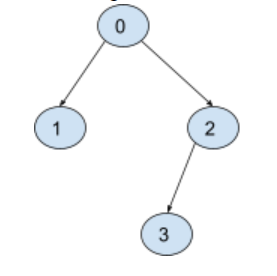New update is available. Click here to update.

# Validate Binary Tree Nodes

Last Updated: 25 Mar, 2021
Difficulty: Moderate

## PROBLEM STATEMENT

#### Example:

``````Let’s say we have n=4 nodes, 'LEFT_CHILD' = {1, -1, 3, -1} and
RIGHT_CHILD = {2, -1, -1, -1}. So the resulting tree will look like this:
````````````It will return True as there is only one valid binary tree and each node has only one parent and there is only one root.
``````
##### Input format:
``````The very first line of input contains an integer ‘T’ denoting the
number of test cases.

The first line of every test case contains an integer ‘N’ denoting
the number of nodes of the tree.

The next two lines of every test case contain the 'LEFT_CHILD' array and
'RIGHT_CHILD' array respectively.
``````
##### Output format:
``````For each test case, return either ‘Yes’ or ‘No’ that whether from given nodes, we can form exactly one valid binary tree or not.

Output for each test case is printed on a separate line.
``````
##### Note:
``````You do not need to print anything, it has already been taken care of. Just return ‘True’ or ‘False’ that whether from given nodes we can form exactly one valid binary tree or not.

The nodes have no values and that we only use the node numbers in this problem.
``````
##### Constraints:
``````1 <= T <= 10
1 <= N <= 10^4
LEFT_CHILD.length == RIGHT_CHILD.length == N
-1 <= LEFT_CHILD[i], RIGHT_CHILD[i] <= N - 1

Time Limit: 1 sec
``````## Approach 1

Approach:

• In this approach, we have to check three things:
• The count of the parent of each node should one
• The count of the possible root should be one and if there exists a possible root
• Connected components should be equal to one.
• We will keep the count of the parent and if any node has more than one parent we will return False.
• Then we will check for the possible root and if the count of possible root id greater than one then we will return False.
• Then we will unite the nodes in a tree through a disjoint data structure and find the total number of connected components. If its count is greater than one then we will return False.
• If all the above conditions are met then we will return True.
• Refer to this doc for disjoint set union https://cp-algorithms.com/data_structures/disjoint_set_union.html

Algorithm:

• First, create an array ‘NODE_PARENT’ to store the count of the parent of each node.
• Next traverse over the ‘LEFT_CHILD’ array and if LEFT_CHILD[i] is not equal to -1 then increase the parent of LEFT_CHILD[i] by one i.e do NODE_PARENT[LEFT_CHILD[i]] += 1. Also check if NODE_PARENT[LEFT_CHILD[i]] > 1 then return ‘False’.
• In the same traversal do same steps for the ‘RIGHT_CHILD’ array also.
• No, take two variables POSSIBLE_ROOT initialised to -1 and COUNT_ROOT = 0.
• Now traverse again from i = 0 to i = N and check if there is any node with a parent equal to 0.
• If NODE_PARENT[i] = 0 then POSSIBLE_ROOT = i and COUNT_ROOT  = COUNT_ROOT + 1.
• After traversal check if COUNT_ROOT > 1 or POSSIBLE_ROOT = -1 then return ‘False’.
• Now traverse again from i = 0 to i = N and unite the nodes using Disjoint Set Union in order to find the connected components.
• Take ROOT = i, LEFT = LEFT_CHILD[i] and RIGHT = RIGHT_CHILD[i]
• Unite ROOT with LEFT if LEFT_CHILD[i] is not equal to -1.
• Unite ROOT with RIGHT if RIGHT_CHILD[i] is not equal to -1.
• Now if connected components are greater than one then return ‘False’.
• Otherwise finally return ‘True’ if all the above conditions are met.